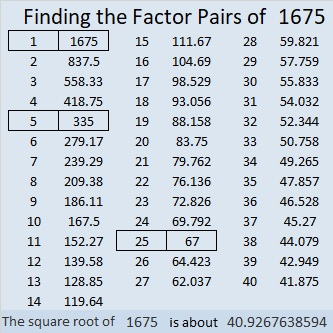# 1675 You CAN Solve This Level 3 Puzzle!

Great news for math enthusiasts, students, and teachers everywhere! The #148 Playful Math Education Carnival was published today at Math Book Magic!

Contents

### Today’s puzzle:

I am confident that you can solve this level 3 puzzle! Here’s how: Using only the numbers from 1 to 10, write the factors of 27 and 6 in the appropriate cells. Next, write 18’s factors. Then, since this is a level 3 puzzle, write the factors of 30, 50, 40, 36, 14, 56, and 8, in that order, until all the numbers from 1 to 10 appear in the first column as well as in the top row. As always, there is only one solution.What did I tell you? You could solve it!

### Factors of 1675:

• 1675 is a composite number.
• Prime factorization: 1675 = 5 × 5 × 67, which can be written 1675 = 5² × 67.
• 1675 has at least one exponent greater than 1 in its prime factorization so √1675 can be simplified. Taking the factor pair from the factor pair table below with the largest square number factor, we get √1675 = (√25)(√67) = 5√67.
• The exponents in the prime factorization are 2 and 1. Adding one to each exponent and multiplying we get (2 + 1)(1 + 1) = 3 × 2 = 6. Therefore 1675 has exactly 6 factors.
• The factors of 1675 are outlined with their factor pair partners in the graphic below.### More About the Number 1675:

Did you notice that 1675 = 5 × 67 × 5?

How many quarters are in \$16.75?
Well, 17 × 4 quarters = 68 quarters = \$17.00.
Subtracting one quarter from both sides of the equation, we get
67 quarters = \$16.75.

1675 is the hypotenuse of TWO Pythagorean triples:
469-1608-1675, which is (7-24-25) times 67, and
1005-1340-1675, which is (3-4-5) times 335.

1675 is the difference of two squares in THREE different ways:
838² – 837² =  1675,
170² – 165² =  1675, and
46² – 21²  =  1675.

This site uses Akismet to reduce spam. Learn how your comment data is processed.